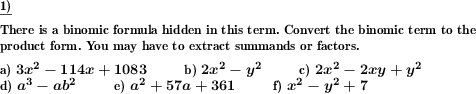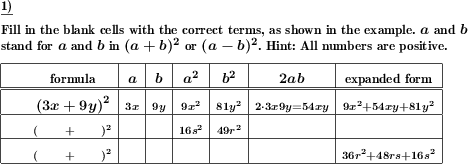Custom math worksheets at your fingertips# Details for problem "Expand and simplify binomic formula, advanced"

Quickname: 7517

Junior High School, Middle School, High School.

## Summary

Apply binomic formula expansion rules to simplify term. Advanced version mit multiples of variables as summands.

## Example## Description

Apply the well known binomic formula expansion rules to expand and simplify terms.

The summands of the formula's factors are multiples of a variable.

The number of problems is selectable. For the types of formulas that will appear it can be specified that only some of the three binomic formulas will be used.

Variables that appear will always be named a and b or x and y.

Download free printable worksheets for this math problem here. The worksheet contains the problems only, the solution sheet includes the answers. Just click on the respective link.

•Worksheet 1Solution sheet with answers
•Worksheet 2Solution sheet with answers
•Worksheet 3Solution sheet with answers

If you can not see the solution sheets for download, they may be filtered out by an ad blocker that you may have installed. If this is the case, please allow ads for this page and reload the page. The solution sheets will then reappear.

• Do these sample worksheets do not really fit?
• Do you need more math worksheets, with a different level of difficulty?
• Would you like to combine different problems on a worksheet and adjust them to your needs?
• As a teacher, you can put together your own worksheets using the automatically generated math problems provided.
With a free initial credit, you can start creating your own math worksheets in a few minutes.

You can try it for free! Register here, to create custom worksheets now!

## Customization options for this problem

Parameter
Possible values
Number of problems
1, 2, 3, 4, 5, 6, 7, 8, 9, 10
Binomic formula types
(a+b)^2, (a-b)^2, (a+b)(a-b), (a+b)^2 & (a-b)^2, all
Negative factors allowd
Yes, No

## Similar problems

Remark
Description
Get to know the elements of binomic formulas
Elements of a binomic formula and their expanded form are grouped in a table. Fill the empty spaces.
More complex version
Apply binomic formula expansion rules to simplify term.

## dw-Math worksheet templates that do contain this problem

Name
Title
Description
Use the binomic formulas to simplify and expand terms.

## Other types of problems that appear on worksheets with this problem:

Relevance
Name
Description
Quickname
Example
****
Extract binomic formula from term
A binomic formula is hidden in a term. Factor out the binomic formular or extract the summand.****
Simplify term with binomic formula, combine like terms
Simplify a term which contains a binomic formula****
Examine the elements of binomic formulas
Elements of a binomic formula and their expanded form are grouped in a table. Fill the empty spaces.